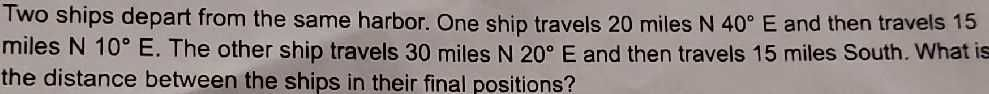### Still have math questions?Two ships depart from the same harbor. One ship travels $$20$$ miles $$N 40 ^ { \circ } E$$ and then travels $$15$$ miles $$N 10 ^ { \circ } E$$ . The other ship travels $$30$$ miles $$N 20 ^ { \circ } E$$ and then travels $$15$$ miles South. What is the distance between the ships in their final positions?